Courses

# Test: Exponents And Powers - 3

## 20 Questions MCQ Test Mathematics (Maths) Class 7 | Test: Exponents And Powers - 3

Description
This mock test of Test: Exponents And Powers - 3 for Class 7 helps you for every Class 7 entrance exam. This contains 20 Multiple Choice Questions for Class 7 Test: Exponents And Powers - 3 (mcq) to study with solutions a complete question bank. The solved questions answers in this Test: Exponents And Powers - 3 quiz give you a good mix of easy questions and tough questions. Class 7 students definitely take this Test: Exponents And Powers - 3 exercise for a better result in the exam. You can find other Test: Exponents And Powers - 3 extra questions, long questions & short questions for Class 7 on EduRev as well by searching above.
QUESTION: 1

### 23+23+23+23 is equal to ____.

Solution:

We have
23+23+23+2= 4 × (23
= 2× 2= 25

QUESTION: 2

### The value of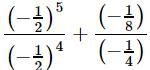is ____.

Solution: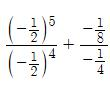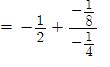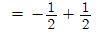= 0

QUESTION: 3

### {(1/3)−3−(1/2)−3} ÷ (1/4)−3 ?

Solution:

{(1/3)−3−(1/2)−3} ÷ (1/4)−3 = {(3)3−(2)3} ÷ (4)3 = {27−8} ÷ 64 = 19/64

QUESTION: 4

The value of (−8) × (−8) × (−8) × (−8) × (−8) + (−8) × (−8) × (−8) × (−8) × (−8) is ____.

Solution:

(−8)5+ (−8)= −2×(8)5 = −2×(2)15

= −(2)16 = −(4)8

QUESTION: 5

If (5/3)−5 × (5/3)11 = (5/3)8+x, then x = ?

Solution:

(5/3)−5 × (5/3)11 = (5/3)8+x
⇒ (5/3)−5+11 = (53)8+x

⇒ (5/3)6 = (5/3)8+
On comparing, we get,
8 + x = 6

⇒ x = −2

QUESTION: 6

The value of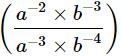is ________.

Solution:=(a−2+3 × b−3+4)

= a×b

= ab

QUESTION: 7

{(3/4)−1−(1/4)−1}−1 = ?

Solution:

{(3/4)−1−(1/4)−1}−1 = {4/3−4}−1 = {−8/3}−1=−3/8

QUESTION: 8

Express the given number using exponential notation: 3125

Solution:

3125 = 5 × 5 × 5 × 5 × 5 = 55

QUESTION: 9

If (5/9)4 × (5/9)−10 = (5/9)−4(5/9)2a−1,then the value of a is _____.

Solution: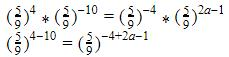Equating the powers,
-6=2a-5
2a=-1
a=-1/2

QUESTION: 10

Which of the following is equivalent to 7.7×10−6 ?

Solution:

7.7×10−6 = 7.7/100000 = 0.0000077

QUESTION: 11

If (3/2)2 × (3/2)a+5 = (3/2)8, then a = ____.

Solution:

(3/2)× (3/2)a+5 = (3/2)8
⇒(3/2)a+7 = (3/2)8
On comparing, we get,
a+7 = 8 ⇒ a =1

QUESTION: 12

The number which is multiplied by (−8)−1 to obtain a product equal to 10−1 is ____.

Solution:

Let the number be x, Then, (−8)−1× x = (10)−1

⇒(−1/8) × x = (1/10) ⇒ x= −4/5

QUESTION: 13

The value of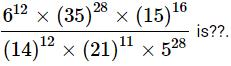Solution:

Explanation :  [(2*3)12 * (5*7)28 * (5*3)16]/[(7*2)12 * (7*3)11 * 528]

= [(2)12 * (3)12 * (5)28 * (7)28 * (5)16 * (3)16]/[(7)12 * (2)12 * (7)11 * (3)11 * (5)28]

= [(2)12 * (3)12+16 * (5)28+16 * 316]/[(7)12+11 * (2)12 * (3)11 * (5)28]

= [(2)12 * (3)28 * (5)44 * 316]/[(7)23 * (2)12 * (3)11 * (5)28]

= 317 * 516 * 75

QUESTION: 14

The value of a− ba, if a = 3, b = 7 is

Solution:

ab − ba =37−7= 2187−343 = 1844

QUESTION: 15

The value of (23/25)× (−1/2)×2× (3/4)2 is

Solution:

(23/25)× (−1/2)×2× (3/4)2

=1×(−1)× (2)−5 × 2× 3× (2)−4=−9/64

QUESTION: 16

A rectangular piece of land is to be sold off in smaller pieces. The total area of the land is 217 sq. miles. The pieces to the cut out are 162 sq. miles in size. How many smaller pieces of the land can be sold at the given size?

Solution:

Required number of pieces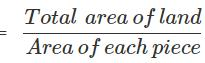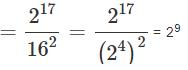QUESTION: 17

Pooja spends about Rs.40000 each month on utensils hold. If she has about 1×10utensils, what is the total amount of money (in Rs.) spent on the households in one month?

Solution:

Total amount of money spent Rs.(40000×1×108) = Rs.(4.0×1012)

QUESTION: 18

All the planets revolve around the Sun in elliptical orbits. Uranus's farthest distance from the Sun is approximately 3.004×109 km and its closest distance is approximately 2.7499×109 kmkm. What is the average distance of Uranus from the Sun?

Solution:

Distance of Uranus from Sun =(3.004×109)km
Uranus closest distance from Sun =(2.7499×109)km
Total distance = 3.004×10+ 2.7499×109
= (5.753×109)km
Average distance = (5.753×109)/2
= (2.8765×109)km

QUESTION: 19

There are three places A, B, C in a straight line as shown below. If distance between place A and B is (2.4×106)m and distance between 8 and C is (5.2×105)m, then find the distance between place A and C in standard form.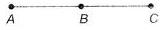Solution:

Distance between place A and B
= 2.4×106m = 2400000 m
Distance between place 6 and C = 5.2×105 m = 5.2×100000 m = 520000 m
Distance between place A and C
= (2.4×10+ 5.2×105)m = (2400000 + 520000)m = 292×104m
Standard form of 292×104m = 2.92×106

QUESTION: 20

Mr Gupta asked two of his students to solve the expression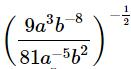and write the answer on blackboard. Aryan wrote the answer as 3a−4bwhereas Ayan wrote the answer as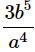. Who wrote the correct answer?

Solution:

We have,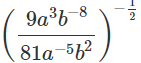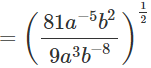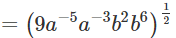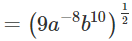= 3a−4b5